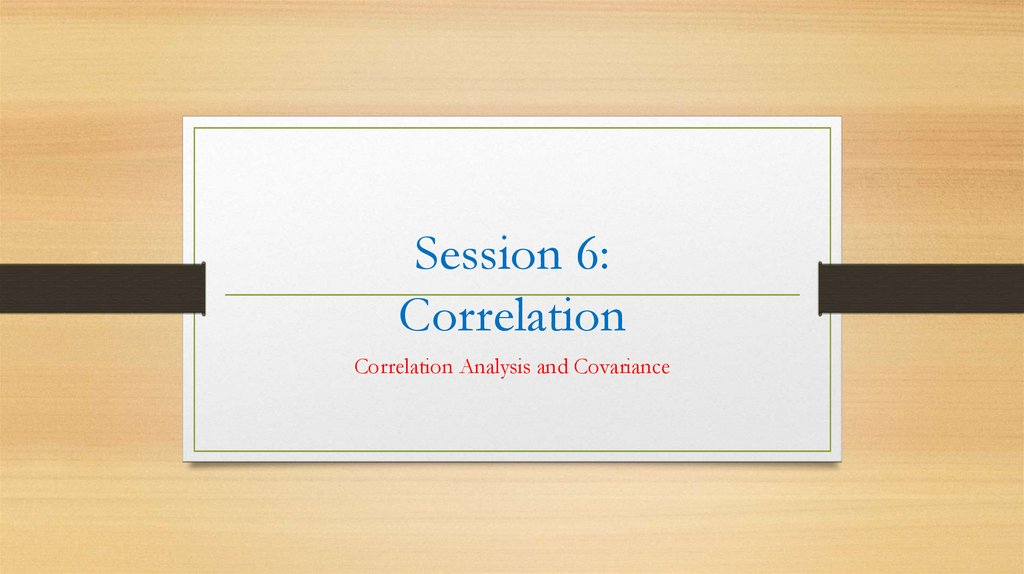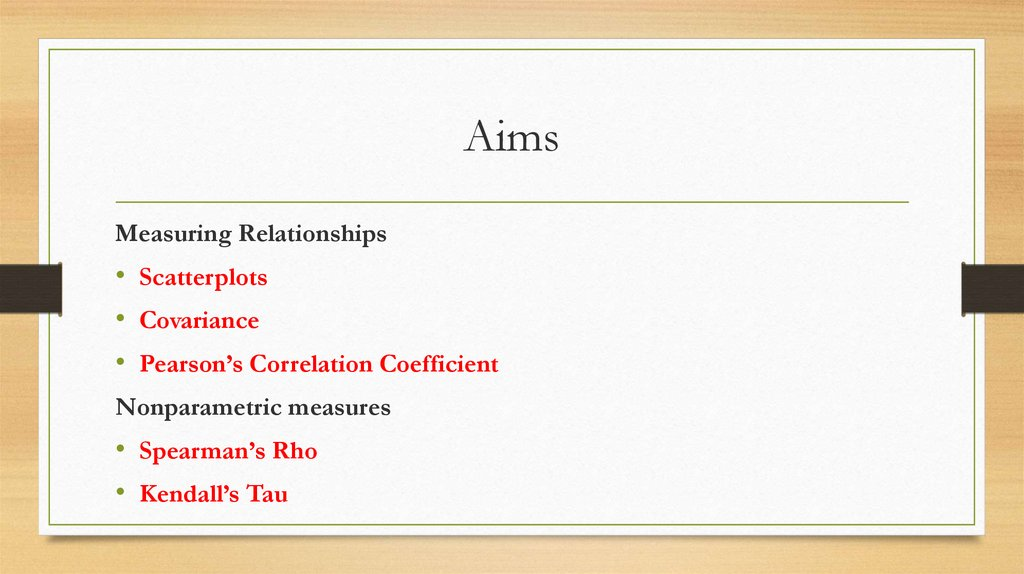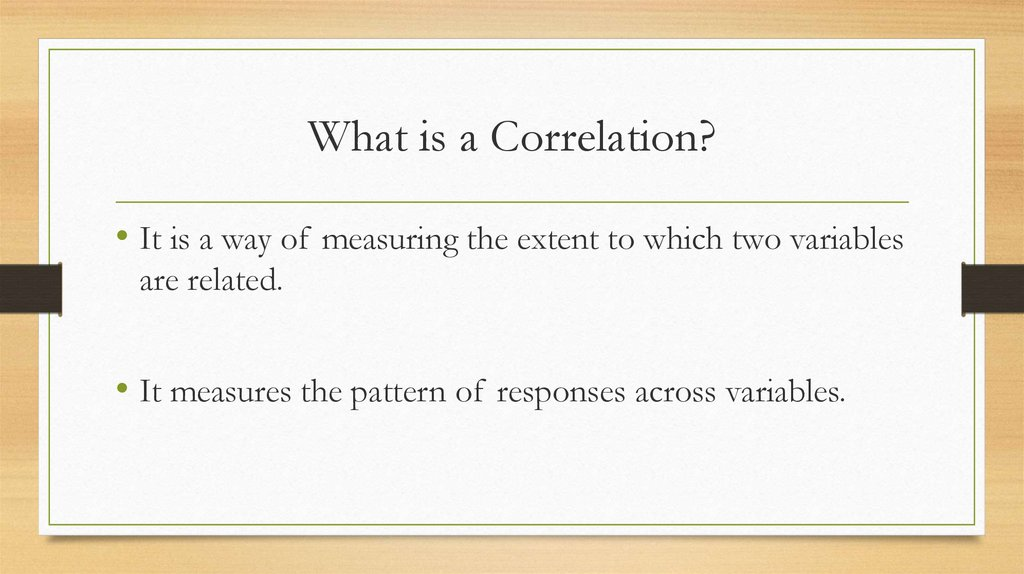# Correlation Analysis and Covariance

## 1. Session 6: Correlation

Correlation Analysis and Covariance

## 2. Aims

Measuring Relationships
• Scatterplots
• Covariance
• Pearson’s Correlation Coefficient
Nonparametric measures
• Spearman’s Rho
• Kendall’s Tau

## 3. What is a Correlation?

• It is a way of measuring the extent to which two variables
are related.
• It measures the pattern of responses across variables.

## 4. Measuring Relationships

• We need to see whether as one variable increases, the
other increases, decreases or stays the same.
• This can be done by calculating the Covariance.

## 5. Covariance

• Calculate the error between the mean and each subject’s
score for the first variable (x).
• Calculate the error between the mean and their score for the
second variable (y).
• Multiply these error values.
• Add these values and you get the cross product deviations.
• The covariance is the average cross-product deviations:

## 6. Problems with Covariance

It depends upon the units of measurement.
• E.g. The Covariance of two variables measured in Miles might be 4.25, but if the
same scores are converted to Km, the Covariance is 11.
One solution: standardize it!
• Divide by the standard deviations of both variables.
The standardized version of Covariance is known as the Correlation coefficient.
• It is relatively affected by units of measurement.

## 9. Things to know about the Correlation

It varies between -1 and +1
• 0 = no relationship
Coefficient of determination,